• 0.相关公式 在笛卡尔坐标系上，一个标准的是这样的： 已知圆心坐标 （x0，y0），半径 R，角度 a，则圆边上点（x'，y'）的坐标为： C 的三角函数参数为弧度，转换如下： 角度转弧度： 弧度转角度： 但...
（2021-2-11 之前把笛卡尔坐标的 x 和 y 标注反了，已修正）

0.圆相关公式

在笛卡尔坐标系上，一个标准的圆是这样的：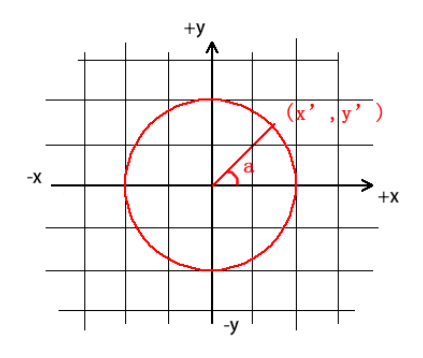已知圆心坐标 （x0，y0），半径 R，角度 a，则圆边上点（x'，y'）的坐标为：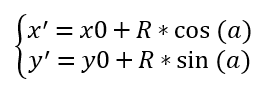C 的三角函数参数为弧度，转换如下：

角度转弧度：

弧度转角度：

但我们知道，Qt 绘图是屏幕坐标系，起点在左上角，以右下角为正方向：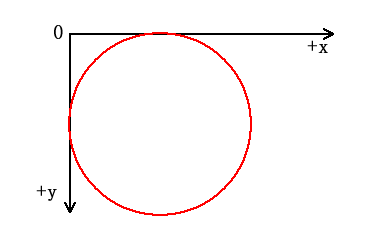（可以把计算后的 y 取反来得到想要的效果）

void MainWindow::paintEvent(QPaintEvent *event)
{
event->accept();

QPainter painter(this);
painter.setPen(QPen(Qt::red,2));
//移动坐标中心点到窗口中心，默认左上角为起点，往右下为正方向
painter.translate(width()/2,height()/2);
//画一个圆，圆心为起点（上一步移动到的正中），半径100px
const int R=100;
painter.drawEllipse(QPoint(0,0),R,R);
//计算45度角圆边上的点，角度需要转换为弧度
const int x=0+R*cos(a);
const int y=0+R*sin(a);
//因为屏幕坐标系y轴正方向和笛卡尔坐标系相反，所以y取反就是我们要的结果了
painter.drawLine(QPoint(0,0),QPoint(x,-y)); //y取反
}

运行结果：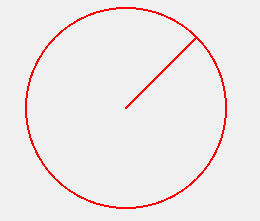1.椭圆相关公式

在笛卡尔坐标系上，一个标准的椭圆是这样的：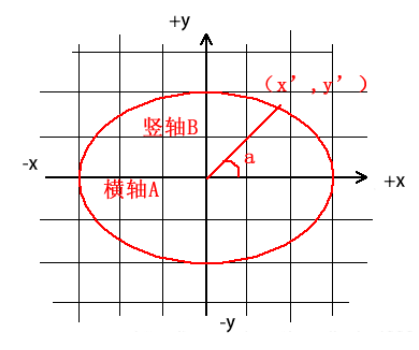已知圆心坐标 （x0，y0），横轴 A（长半轴），竖轴 B（短半轴），角度 a，则圆边上点（x'，y'）的坐标为：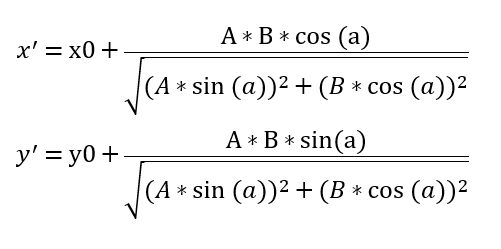椭圆半径：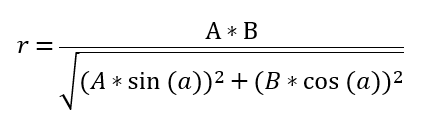（可以发现，与普通圆相比，不考虑椭圆角度的话只是半径变化了，其他部分是一样的）

void MainWindow::paintEvent(QPaintEvent *event)
{
event->accept();

QPainter painter(this);
painter.setPen(QPen(Qt::red,2));
//移动坐标中心点到窗口中心，默认左上角为起点，往右下为正方向
painter.translate(width()/2,height()/2);
//画一个圆，圆心为起点（上一步移动到的正中），半径100px
const int A=150; //横轴
const int B=100; //竖轴
painter.drawEllipse(QPoint(0,0),A,B);
//计算45度角圆边上的点，角度需要转换为弧度
const int R=A*B/sqrt(pow(A*sin(a),2)+pow(B*cos(a),2)); //计算对应角度的半径
const int x=0+R*cos(a);
const int y=0+R*sin(a);
//因为屏幕坐标系y轴正方向和笛卡尔坐标系相反，所以y取反就是我们要的结果了
painter.drawLine(QPoint(0,0),QPoint(x,-y)); //y取反
}

运行结果：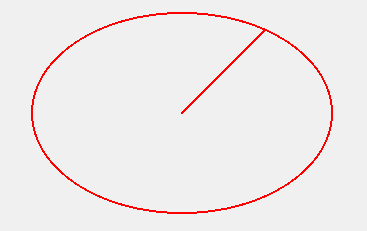2.旋转矩阵

上面的角度都是从右侧开始，椭圆也是标准椭圆，如果我们想旋转一定角度来绘制，就需要用到我们的旋转矩阵了。

（本节主要来自参考博客）

已知 A(x,y) ，求旋转 a 角度后的 B(x’,y’) 坐标：公式推导：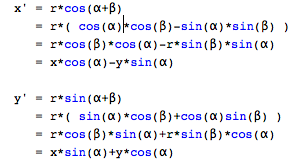根据矩阵乘法计算规则，可以推出：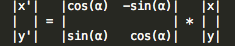操作流程：

把图形的各点平移，令旋转中心平移至原点；
乘以旋转矩阵；
再平移至原来的旋转中心。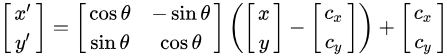我们将上面椭圆的代码改一下，逆时针旋转 45 度：


void MainWindow::paintEvent(QPaintEvent *event)
{
event->accept();

QPainter painter(this);
painter.setPen(QPen(Qt::red,2));
//移动坐标中心点到窗口中心，默认左上角为起点，往右下为正方向
painter.translate(width()/2,height()/2);
//画一个圆，圆心为起点（上一步移动到的正中），半径100px
const int A=150; //横轴
const int B=100; //竖轴
painter.rotate(-45); //qpainter是顺时针转的
painter.drawEllipse(QPoint(0,0),A,B);
painter.rotate(45);

//计算45度角圆边上的点，角度需要转换为弧度
const int R=A*B/sqrt(pow(A*sin(a),2)+pow(B*cos(a),2)); //计算对应角度的半径
const int x=0+R*cos(a);
const int y=0+R*sin(a);
//根据旋转矩阵计算
const int x2=x*cos(a2)-y*sin(a2);
const int y2=x*sin(a2)+y*sin(a2);
//因为屏幕坐标系y轴正方向和笛卡尔坐标系相反，所以y取反就是我们要的结果了
painter.drawLine(QPoint(0,0),QPoint(x2,-y2)); //y取反
}

运行结果：

（第一次计算的角度坐标是计算标准椭圆下的位置，第二次是根据椭圆的旋转角度做旋转得到的坐标）

（本来就有 45 度，再旋转 45 度就成了垂直向上了）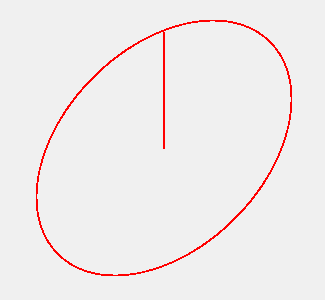（虽然线可以直接 QPainter 旋转的方式绘制，但是这样文本也旋转了）

3.参考

博客（圆和椭圆坐标）：https://blog.csdn.net/xiamentingtao/article/details/85804823

博客（椭圆坐标）：https://blog.csdn.net/chenlu5201314/article/details/99678398

博客（二维旋转矩阵）：https://blog.csdn.net/Mynameisyournamewuyu/article/details/88650409

展开全文QPainter Qt
• 上任意角度的点的坐标 如上，给定圆心（Cx,Cy）,半径为R， 求θ\thetaθ对应的点的坐标？ 此处θ\thetaθ是相对于水平轴的角度。 显然我们可以使用极坐标转换来求： {px=Cx+Rcos(θ)py=Cy+Rsin(θ) \left\{\...
圆上任意角度的点的坐标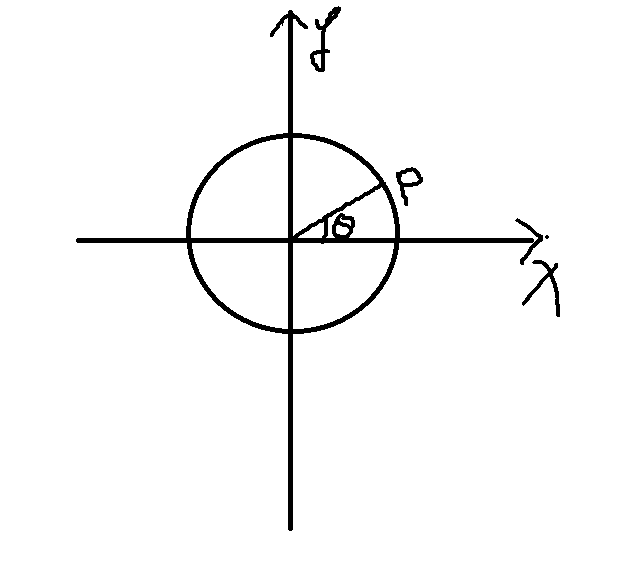如上图，给定圆心（Cx,Cy）,半径为R， 求$\theta$对应的点的坐标？ 此处$\theta$是相对于水平轴的角度。
显然我们可以使用极坐标转换来求：
$\left\{\begin{matrix} px= Cx+Rcos(\theta) \\ py= Cy+Rsin(\theta) \end{matrix}\right.$
注意如果以上竖直坐标系向下，此时变为图像的坐标系，即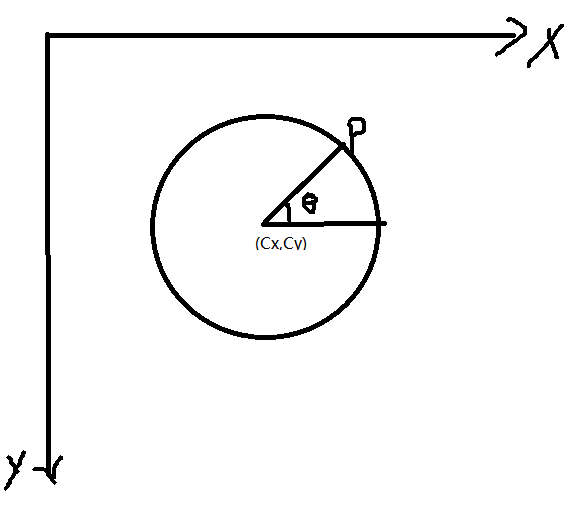结果变成：
$\left\{\begin{matrix} px= Cx+Rcos(\theta) \\ py= Cy-Rsin(\theta) \end{matrix}\right.$
仅仅是y变了。
椭圆上任意角度的点的坐标
首先我们先来考虑标准椭圆上任意角度的点的坐标，再进行推广。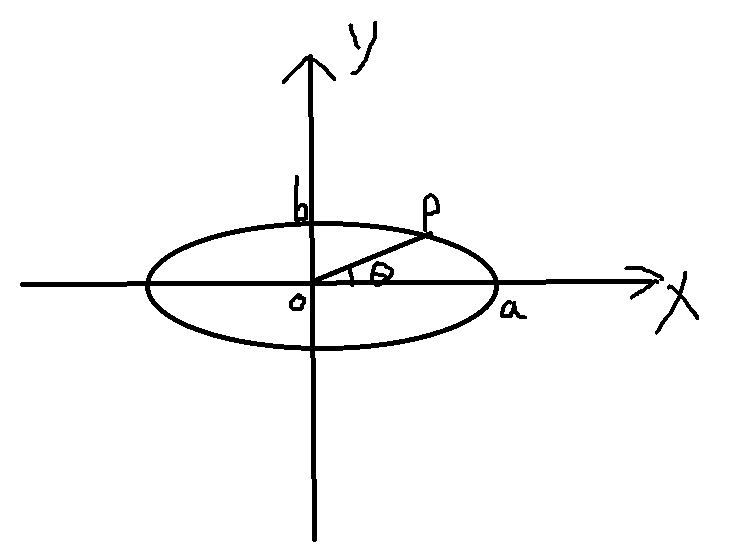已知主轴a（即椭圆箭头所指方向对应的轴）,次轴b,求解与水平轴相交$\theta$对应的点的坐标？
$\left\{\begin{matrix} \frac{x^2}{a^2}+\frac{y^2}{b^2}&=&1 \\ \frac{y}{x}& =& \tan(\theta) \end{matrix}\right.$
将上面的第二式带入第一式，可以求解得到：
$x^2=\frac{a^2b^2}{b^2+a^2\tan^2(\theta)}$
这时我们考虑$\theta$的范围，可以得到：
(一). $0\leq\theta<pi/2$ 或者 $\frac{3*pi}{2}<\theta \leq 2*pi$
$\left\{\begin{matrix} x=\frac{ab}{\sqrt{b^2+a^2\tan^2(\theta)}} \\ y=\frac{ab\tan(\theta)}{\sqrt{b^2+a^2\tan^2(\theta)}} \end{matrix}\right.$
(二). $pi/2<\theta<\frac{3*pi}{2}$
$\left\{\begin{matrix} x=-\frac{ab}{\sqrt{b^2+a^2\tan^2(\theta)}} \\ y=-\frac{ab\tan(\theta)}{\sqrt{b^2+a^2\tan^2(\theta)}} \end{matrix}\right.$
(三).  $\theta = pi/2$
$\left\{\begin{matrix} x=0 \\ y=b \end{matrix}\right.$
(四).  $\theta = \frac{3*pi}{2}$
$\left\{\begin{matrix} x=0 \\ y=-b \end{matrix}\right.$
再考虑一般情况下的椭圆，如下：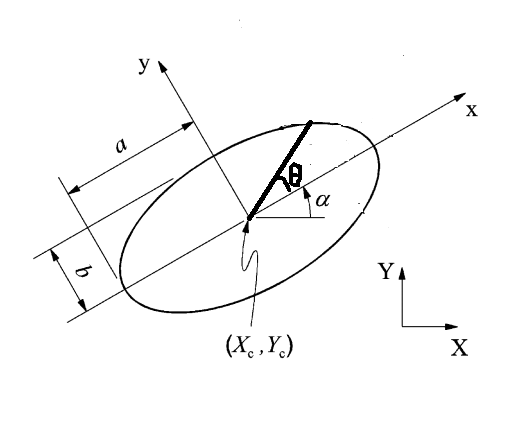如上$\theta\in[0,2*pi]$是相对于主轴的角度，$\alpha\in[-pi,pi]$, 不过我们一般仅仅考虑$\alpha\in[0,pi]$,求$\theta$对应的点的坐标？
基本思路就是先转换成标准椭圆，再应用标准椭圆下的结果。
显然我们需要将以上椭圆的中心移到原点，再绕原点旋转$-\alpha$ ,即顺时针旋转$\alpha$，即：
$\begin{pmatrix} x\\ y \end{pmatrix}=R(\begin{pmatrix} X\\ Y \end{pmatrix}-\begin{pmatrix} X_c\\Y_c \end{pmatrix})$
其中
$R=\begin{pmatrix} cos(-\alpha) &-sin(-\alpha) \\ sin(-\alpha) & cos(-\alpha) \end{pmatrix}=\begin{pmatrix} cos(\alpha) &sin(\alpha) \\ -sin(\alpha) & cos(\alpha) \end{pmatrix}$
因此
$\begin{pmatrix} X\\ Y \end{pmatrix} =R^{-1}\begin{pmatrix} x\\ y \end{pmatrix}+\begin{pmatrix} X_c\\Y_c \end{pmatrix}$
其中
$R^{-1}=R^{T}=\begin{pmatrix} cos(\alpha) &-sin(\alpha) \\ sin(\alpha) & cos(\alpha) \end{pmatrix}~~~~~~~~~~~~~~~~~~~~~~~~~~~(\bigstar)$
这样将前面$\begin{pmatrix} x\\ y \end{pmatrix}$ 的四个结果应用过来，即得到倾斜的椭圆上的对应角度的点的坐标。
扩展：

如果以上的角度是相对于水平轴的角度，则对应的椭圆上的点的坐标如何求呢？
答： 其实很简单，只需要：
$\theta'=\left\{\begin{matrix} \theta-\alpha+2*pi, & \theta<\alpha \\ \theta-\alpha, & \theta \geq \alpha \end{matrix}\right.$
将$\theta'$替换以上标准椭圆下的$\theta$即可。
如果竖直坐标轴为竖直向下的，即为图像坐标系下的椭圆，那么如何求对应的角度？
答：
需要做两方面的改变即可：
$\left\{\begin{matrix} \alpha\rightarrow -\alpha\\ y\rightarrow -y \end{matrix}\right.$
第一行改变$\bigstar$出的$\alpha$. 第二行改变标准椭圆下的y值的符号。

matlab代码
demo.m
################################
center_x=282;
center_y=263;
phi=pi/6;
R1=141;
R2=62;
%DrawEllipse([center_x,center_y],R1,R2,phi);
axis equal;
hold on;
set(gca,'ydir','reverse')
for angle=linspace(0,3*pi/2,360)
[ px,py ] = get_points_ellipse(center_x, center_y,phi,R1,R2, angle );
plot(px,py,'ro');
hold on;
end
axis([0,500,0,500])

function [ px,py ] = get_points_ellipse(center_x, center_y,phi,R1,R2, angle )
% 求椭圆某个角度上的点的坐标。
%https://math.stackexchange.com/questions/22064/calculating-a-point-that-lies-on-an-ellipse-given-an-angle
%https://blog.csdn.net/xiamentingtao/article/details/54934467

%https://stackoverflow.com/questions/17762077/how-to-find-the-point-on-ellipse-given-the-angle
%另一种解法  从极坐标的角度出发。

% 椭圆参数  圆心 (center_x.center_y) 角度 phi(-pi,pi)，实际上仅考虑（0，pi） 沿着角度的主轴半径R1，另一主轴对应的半径R2
% 求相对于水平轴x轴上angle（基于0-2pi之间）上对应的点坐标。
% 注意求解的坐标系为：水平向右为x轴,竖直向上为y轴。 如果竖直向下为y轴，则下面的y应该变换成-y， phi变成-phi

%先将斜的椭圆转正，并平移到原点。方法就是平移到中心，且旋转-phi角度,再应用标准椭圆求取点。
if(angle<phi)
theta = angle-phi+2*pi;  %想对于主轴的角度
else
theta = angle-phi;
end
% theta为相对于主轴的角度
alpha = -phi;    %与竖直的轴有关，如果是竖直向上，则变为正phi
% 先计算标准椭圆 x^2/R1^2+y^2/R2^2=1  与 直线 y/x=tan(angle)的交点。
assert(angle>=0 && angle<=2*pi);
if(theta==pi/2)
x=0;
y=R2;
elseif(theta==pi*3/2)
x=0;
y=-R2;
elseif(theta>pi/2 && theta<pi*3/2)
x=-R1*R2/sqrt(R2^2+R1^2*tan(theta)^2);
y=-R1*R2*tan(theta)/sqrt(R2^2+R1^2*tan(theta)^2);
else
x=R1*R2/sqrt(R2^2+R1^2*tan(theta)^2);
y=R1*R2*tan(theta)/sqrt(R2^2+R1^2*tan(theta)^2);
end
y=-y;    %与竖直的轴有关，如果是竖直向上，则变为正y
% 将以上结果转换回去
px= cos(alpha)*x-sin(alpha)*y+center_x;
py=sin(alpha)*x+cos(alpha)*y+center_y;

end


function DrawEllipse(C,a,b,alpha)
% DRAWELLIPSE plots an ellipse
%   DrawEllipse(C,a,b,alpha) plots ellipse with center C, semiaxis a
%   and b and angle alpha between a and the x-axis

s=sin(alpha); c=cos(alpha);
Q =[c -s; s c]; theta=[0:0.02:2*pi];
u=diag(C)*ones(2,length(theta)) + Q*[a*cos(theta); b*sin(theta)];
plot(u(1,:),u(2,:));
hold on;
plot(C(1),C(2),'+');


显示结果，起点对应着角度为0。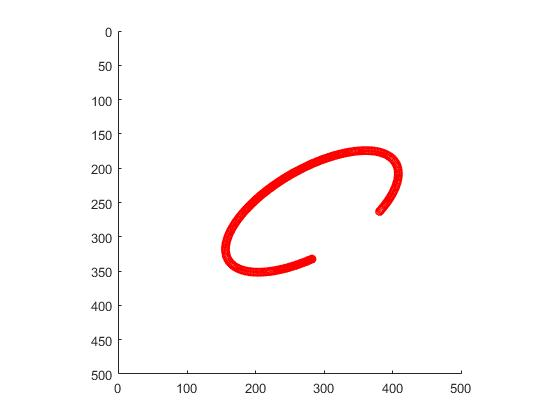另一种基于极坐标变换的高效实现【转载】
转载： https://blog.csdn.net/he_zhidan/article/details/81347426
CDoublePoint2d GetPointOnEllipse(const CDoublePoint2d& ptCenter, double a, double b, double radian, double dChangZhouAngle)
{
double x = a*cos(dLiXin)cos(dChangZhouAngle) - bsin(dLiXin)sin(dChangZhouAngle) + ptCenter.x;
double y = acos(dLiXin)sin(dChangZhouAngle) + bsin(dLiXin)*cos(dChangZhouAngle) + ptCenter.y;
return CDoublePoint2d(x, y);
}
问题：
一，为什么不用atan？
答：atan的范围是[-π/2,π/2]，atan2的范围是(-π,π]。前者只能表示两个象限，后者可以表示4个。
答：这样写，和用atan的效果一样。
参考文献

https://math.stackexchange.com/questions/22064/calculating-a-point-that-lies-on-an-ellipse-given-an-angle （主要参考这个）
https://stackoverflow.com/questions/17762077/how-to-find-the-point-on-ellipse-given-the-angle （这里有一个从极坐标变换角度新的推导）
https://blog.csdn.net/xiamentingtao/article/details/54934467


展开全文椭圆
• 为了分析水平和竖直放置的两种均匀阵的角度模糊特性，基于均匀阵数据模型和方向特性，分别推导了2种阵的模糊角公式根据导出的模糊角公式，系统分析了阵半径、阵元数和入射信号俯仰角对2种阵模糊性的影响...
• 如下，小为椭圆内切，大为椭圆外切。虚线除OE外，全为垂线。 根据椭圆方程，可知E的y坐标 Ey=b∗sint(t)=AyE_y = b*sint\left(t\right)=A_yEy​=b∗sint(t)=Ay​ 又有 tan(t)=BD/ODtan\left(t\right)=BD/ODtan...
计算椭圆上坐标 分两步，求离心角和根据离心角求椭圆上坐标。
椭圆方程
椭圆方程是
$\frac{x^2}{a^2} + \frac{y^2}{b^2} = \left(\frac{x}{a}\right)^2+\left(\frac{y}{b}\right)^2=\left(cos\left(t\right)\right)^2+\left(sin\left(t\right)\right)^2=1$
这个$t$就是离心角的角度。
求离心角
如下图，已知椭圆的角$p$，要求主离心角$t$。如下图，小圆为椭圆内切，大圆为椭圆外切。虚线除OE外，全为垂线。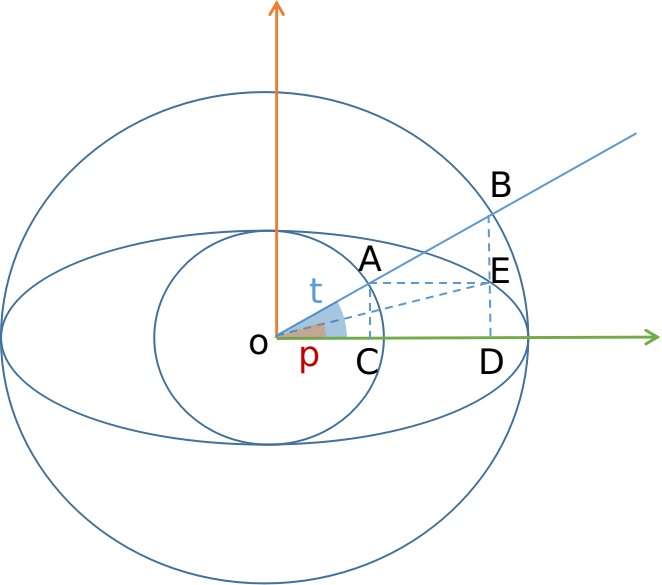根据椭圆方程，可知E的y坐标   $E_y = b*sint\left(t\right)=A_y$
又有
$tan\left(t\right)=BD/OD$   $tan\left(p\right)=ED/OD$
那么 $\frac{tan\left(t\right)}{tan\left(p\right)} = \frac{BD}{ED}=\frac{BD}{AC}=\frac{OD}{OC}=\frac{a}{b}$
所以，$tan\left(t\right)=tan\left(p\right)*\frac{a}{b}$

求解$t$值时，用函数$atan2(x,y)$，这样求出的值是[$-\pi$,$\pi$]，使用$atan$得到的值在[$-\frac{\pi}{2}$,$\frac{\pi}{2}$]

根据离心角求椭圆上给定角度的坐标
根据椭圆公式，可得
$x = a*cos\left(t\right)$ $y = b*sin\left(t\right)$
椭圆出现平移及旋转的情况
这种情况，需要先将椭圆旋转并平移到标准椭圆位置，计算完点坐标再反算回去。如下图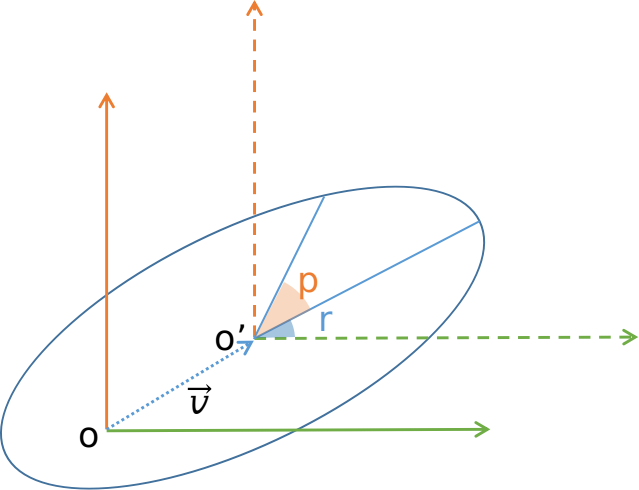旋转后的椭圆坐标与标准椭圆坐标的对应关系如下所示公式：
$\begin{pmatrix}x \\ y\end{pmatrix} = R*\left(\begin{pmatrix}X \\ Y\end{pmatrix}-\begin{pmatrix}X_c \\ Y_c\end{pmatrix}\right)$
其中 ， $R=\begin{pmatrix} cos\left(\gamma \right) & sin\left(\gamma \right) \\ -sin\left(\gamma \right) & cos\left(\gamma \right) \end{pmatrix}$
且  $R^{-1}=R^{T}=\begin{pmatrix} cos\left(\gamma \right) & -sin\left(\gamma \right) \\ sin\left(\gamma \right) & cos\left(\gamma \right) \end{pmatrix}$
那么，旋转后的椭圆上点坐标公式为
$\begin{pmatrix}X \\ Y\end{pmatrix} = R^{-1}*\begin{pmatrix}x \\ y\end{pmatrix} +\begin{pmatrix}X_c \\ Y_c\end{pmatrix}$
最终，
$\begin{array}{c} X= cos\left(\gamma \right)*x -sin\left(\gamma \right)*y = cos\left(\gamma \right)*a*cos\left(t\right) -sin\left(\gamma \right)*b*sin\left(t\right)+X_c \\ Y= sin\left(\gamma \right)*x +cos\left(\gamma \right)*y = sin\left(\gamma \right)*a*cos\left(t\right) +cos\left(\gamma \right)*b*sin\left(t\right)+Y_c \end{array}$
参考：
网页1
网页2


展开全文计算几何
• 任意角度的矩形与圆形的碰撞检测（js）下面介绍的是 无旋转角度的矩形与圆形的碰撞检测：函数ComputeCollision，当相对距离小于圆形半径的时候为碰撞。 参数介绍(w：矩形的宽，h：矩形的高，r：圆形半径，rx：圆形...
任意角度的矩形与圆形的碰撞检测（js）

下面介绍的是 无旋转角度的矩形与圆形的碰撞检测：

函数ComputeCollision，当相对距离小于圆形半径的时候为碰撞。
参数介绍(w：矩形的宽，h：矩形的高，r：圆形半径，rx：圆形中心与矩形中心相对坐标X，ry：圆形中心与矩形中心相对坐标Y）

function ComputeCollision(w, h, r, rx, ry) {
var dx = Math.min(rx, w * 0.5);
var dx1 = Math.max(dx, -w * 0.5);
var dy = Math.min(ry, h * 0.5);
var dy1 = Math.max(dy, -h * 0.5);
return (dx1 - rx) * (dx1 - rx) + (dy1 - ry) *         (dy1 - ry) <= r * r;
}
此代码转载自：http://www.cnblogs.com/kuikui/archive/2012/07/01/2572288.html

此方法仅适用于上图无角度矩形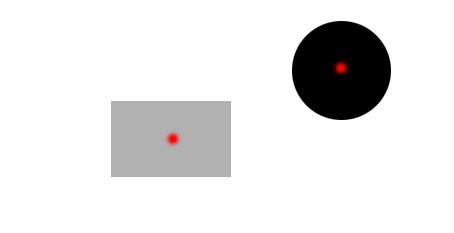当遇到上图这种情况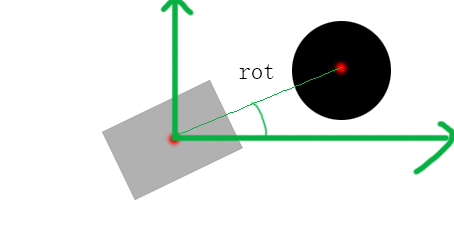我们需要把它转换成能够使用ComputeCollision方法的模型，如上图：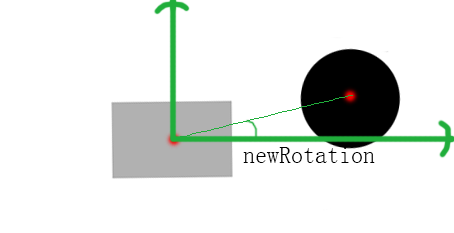原理：让圆与矩形 同时绕着矩形的中心进行旋转，旋转角度为矩形的倾斜角度，然后重新计算rx，ry，（rot是两个中心与X轴的角度，不是矩形的旋转角度）

完整算法代码如下（原创，亲测过）

矩形中心坐标x1,y1 圆形圆心坐标x2,y2 ,rotation 矩形的旋转角度（已知条件）
function ComputeCollision(w, h, r, newrx, newry) {
var dx = Math.min(rx, w * 0.5);
var dx1 = Math.max(dx, -w * 0.5);
var dy = Math.min(ry, h * 0.5);
var dy1 = Math.max(dy, -h * 0.5);
return (dx1 - rx) * (dx1 - rx) + (dy1 - ry) * (dy1 - ry) <= r * r;
}
functuin getNewRx_Ry(){
var json = {};
var distance = twoDistance(x1,y1,x2,y2);
//计算最新角度（与X轴的角度），同数学X Y轴
var newRotation = rot(x1,y1,x2,y2) - rotation;
var newRx = Math.cos(newrot/180*Math.PI) * distance;
var newRy = Math.sin(newrot/180*Math.PI) * distance;
json.newRx = newRx ;
json.newRy = newRy ;
return json;
}

function twoDistance(X1,Y1,X2,Y2) {
return Math.pow((Math.pow((X1 - X2), 2) + Math.pow((Y1 - Y2), 2)), 0.5);
}

function rot(x1,y1,x2,y2){
var value = (y1-y2)/(x1-x2);
return Math.atan(value)*180/Math.PI;
}

///使用
var json = getNewRx_Ry();
var boool = ComputeCollision(w, h, r, json.newRx, json.newRy);

if(boool){console.log('boom')}


展开全文javascript
• 的两种生成算法（角度微分法、Bresenham算法） 文章目录1.角度微分法的原理2.角度微分法的实现（基于matlab）3.Bresenham 算法的原理4.Bresenham 算法的实现（基于matlab） 1.角度微分法的原理 角度微分法是...计算机图形学
• 基于Qt GraphicsView 实现图形的绘制，可以自定义图形绘制在view上。可用于各种图形处理程序中做图形绘制展示和基础测量！
• 本人喜欢用firework，体积小，功能强大。 制作圆角，网上就有现成的在线工具，但是由于工作环境无法上网，所以还是要自己搞搞，...3. 把一个圆角矩形放到图片上， 调整圆角的角度，可以用鼠标拖动黄点调整，也可以在自
• Glide是一个功能强大的图片加载库，下面是平常开发中使用到的一些功能，基于Glide-4.X版本的用法 Glide-github地址 Glide jar包下载地址 加载圆形图片： RequestOptions mRequestOptions = RequestOptions....Glide
• 最近在学习FDDB的人脸识别库，发现他们的标记是用椭圆来标记的，而且还是有角度的椭圆。 在PIL库中好像并没有画出带有角度椭圆的函数，所以在StackOverflow上找到了如下代码： def ellipse_with_angle(im,x,y,major,...脸部识别
• 作图画一个，并标注角度； clc;clear close all R=1; t=0:pi/20:2*pi; x=R*cos(t);y=R*sin(t); plot(x,y),axis equal n=72;a=2*pi/n; % for k=0:n-1 % hold on % plot([-cos(pi+k*a),cos(pi+k*a)],[-sin(pi+k*a),...
• 文章摘要： 上一篇文章，我说了关于php吧文字画在图片上的换行方法，这篇说说项目中图片圆角的处理 我们可能在很多项目中，需要对图片进行圆角...效果如下：如可见，不论是插图，还是文字这背景，我们都做了php 图像处理
• var picview = UIImageView(frame: CGRectMake(X, Y, W, H))  //创建图片视图  picview.layer.masksToBounds = true  //打开图片视图圆角设置 ... //将图片视图圆角度设置为图片视图高ios swift uiimage
• 1. Graphics 绘图画布 Graphics 类相当于一个画布，每个 Swing 组件都通过 Graphics 对象来绘制...绘图的原点位于组件的左上角，如下所示: Graphics类中常用的绘制相关方法： ○ 参数 / 设置: // 创建...java java绘图 Graphics Graphics2D
• 在工作中遇到要在ps中画如两个同心，并且进行6等分。查找资料加自己摸索，可以通过以下方式实现： 1、新建一画布。并用通过标尺画出两条水平和垂直参考线，选择椭圆工具，并在选项设置中选择和从中心两个选项...
• 圆点坐标：(x0,y0)  半径：r  角度：a0  则上任一点为：（x1,y1）  x1 = x0 + r * cos(ao * 3.14 /180 )  y1 = y0 + r * sin(ao * 3.14 /180 )
• 事實上形亦可作為橢之特殊形，因為只要橢之長軸與短軸相等時，即可以作。首先仍然需要宣告下面之指令： axis equal; 繪參數本程式中之繪線，仍採用line的功能，逐點連線繪製。但是連線時係以直線表示...vector c colors plot each
• ## 将椭圆形图像归一化为圆形图像

千次阅读 热门讨论 2013-08-01 10:56:25
% The original image % A 中心在原点的椭圆方程系数 x'Ax = 1 % The coefficients of the ellipse equation centered at the origin % center 椭圆中心 % The center of the ellipse % R 归一化之后的半径 % The ...椭圆 图像 归一化 特征提取
• //的画笔 private Paint paintShortLine; // 短线的画笔 private Paint paintLongLine; //长线的画笔 private int radius = 200; //的半径 private int width = 400 ; //x 中心位置 private int height = ...canvas
• SVG spec 1.2以及之前的...有时候我们需要计算圆弧的圆心和起始角度、结束角度，虽然标准官方文档给出了计算公式的描述，但是没有给出直接的代码。我根据标准文档以及网上的资料，写了一个Javascript函数来做这件事。
• Flutter中的圆角和圆形效果第一种：以图片为容器背景，设置容器四角的圆角角度第二种 ClipRRect 裁剪矩形四角 可自定义圆角度数第三种 ClipOval 直接就是圆形第四种 CircleAvatar拓展 自定义裁剪样式 ClipPath 路径...flutter圆角
• 继承UIView, 自定义一个View 重写方法 - (void)drawRect:(CGRect)rect 完整代码:  - (instancetype)initWithFrame:(CGRect)frame { self = [super initWithFrame:frame]; if (self) { self.backgroundColor
• 一直以来，为IOS添加图片的特殊效果都是通过跟美工的配合，比如，要加阴影，就从美工那边获得一张阴影效果，在界面上画两个UIImageView，将阴影放在下面，图像放上上面，错开一定角度。有比如想做圆角效果，就画...iOS 框架
• 设P1为已知点，与正北的夹角为α， P1转动β角度后到P2，求P2。 设P2与0度角的夹角为Ψ，则Ψ=α+β。 由知 P1(x)=r*sin(α) P1(y)=r*cos(α) P2(x)=r*sin(Ψ)=r*sin(α+β)=r*sin(α)*cos(β)+r*cos(α)*sin...数学 三角函数
• c#雷达扫描 和扇形的背景都有 不用图片都是graphic 画 可以设置线圈 角度等 非常好用 c#雷达扫描 和扇形的背景都有 不用图片都是graphic 画 可以设置线圈 角度等 非常好用
• 1.Smith圆图阻抗匹配计算软件原阻抗匹配计算软件(Smith圆图计算器)是一款计算史密斯圆图的软件，该软件界面简洁，操作方便，体积小巧，功能全面，而且这个做阻抗匹配很好的，但现在还不会用，希望对人家有...
• 彩色圆环统计 事先说明： 如果对View的绘制不熟悉的话，可以先查阅资料绘制简单的几何图形，再来阅读本篇文章 如果是对View刚入门的，可以先从我最简单的View学起：简单实现边缘凹凸电子票效果 更多有趣...控件 自定义View
• 项目中经常用圆形统计来显示收益占比，消费占比等数据，像支付宝账单那样，感觉挺有意思，那么动手来自己撸一个，先来看一下最终效果： 这个东西，想一下思路挺清晰的，就是算一下各个数据的比例，然后根据...android...